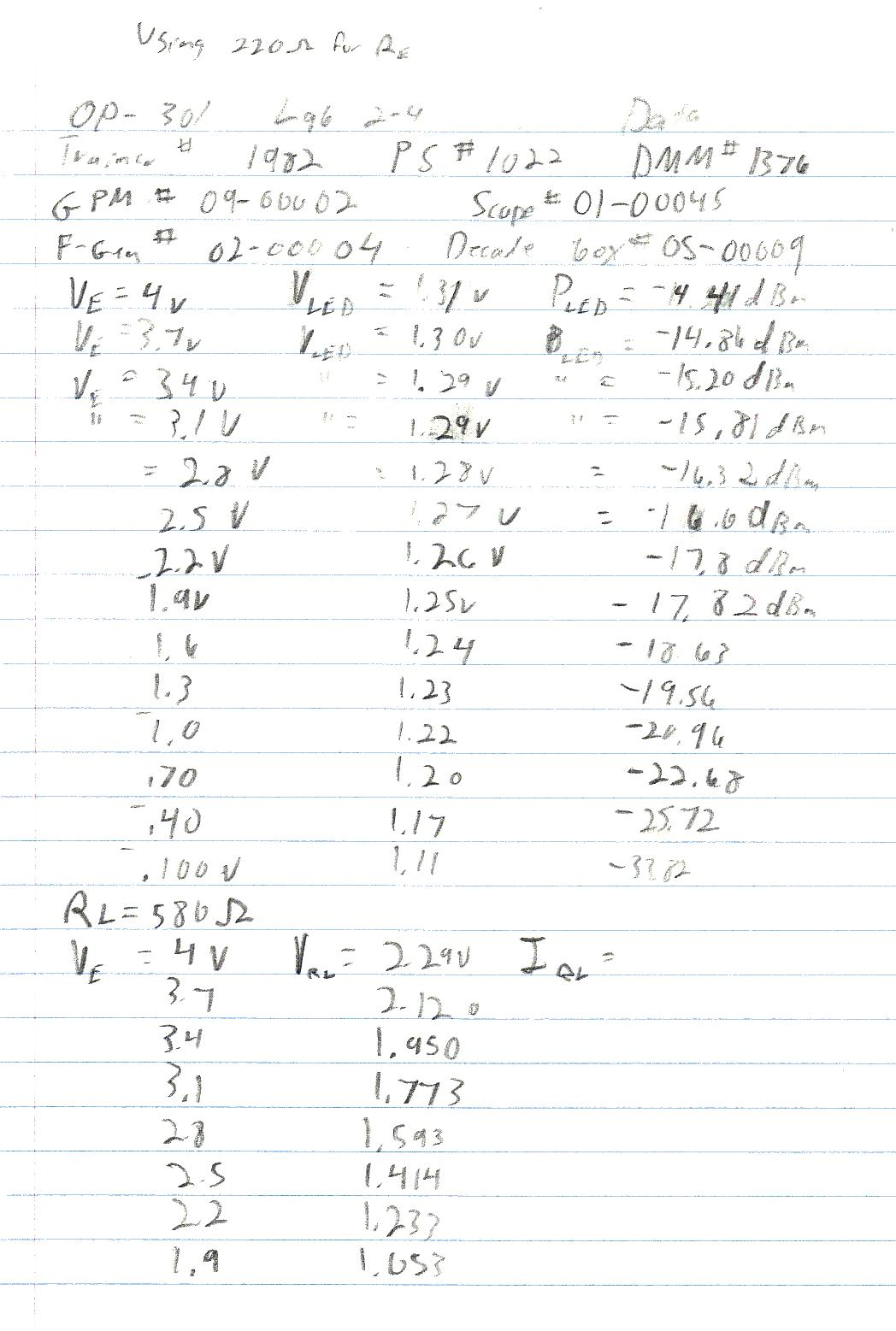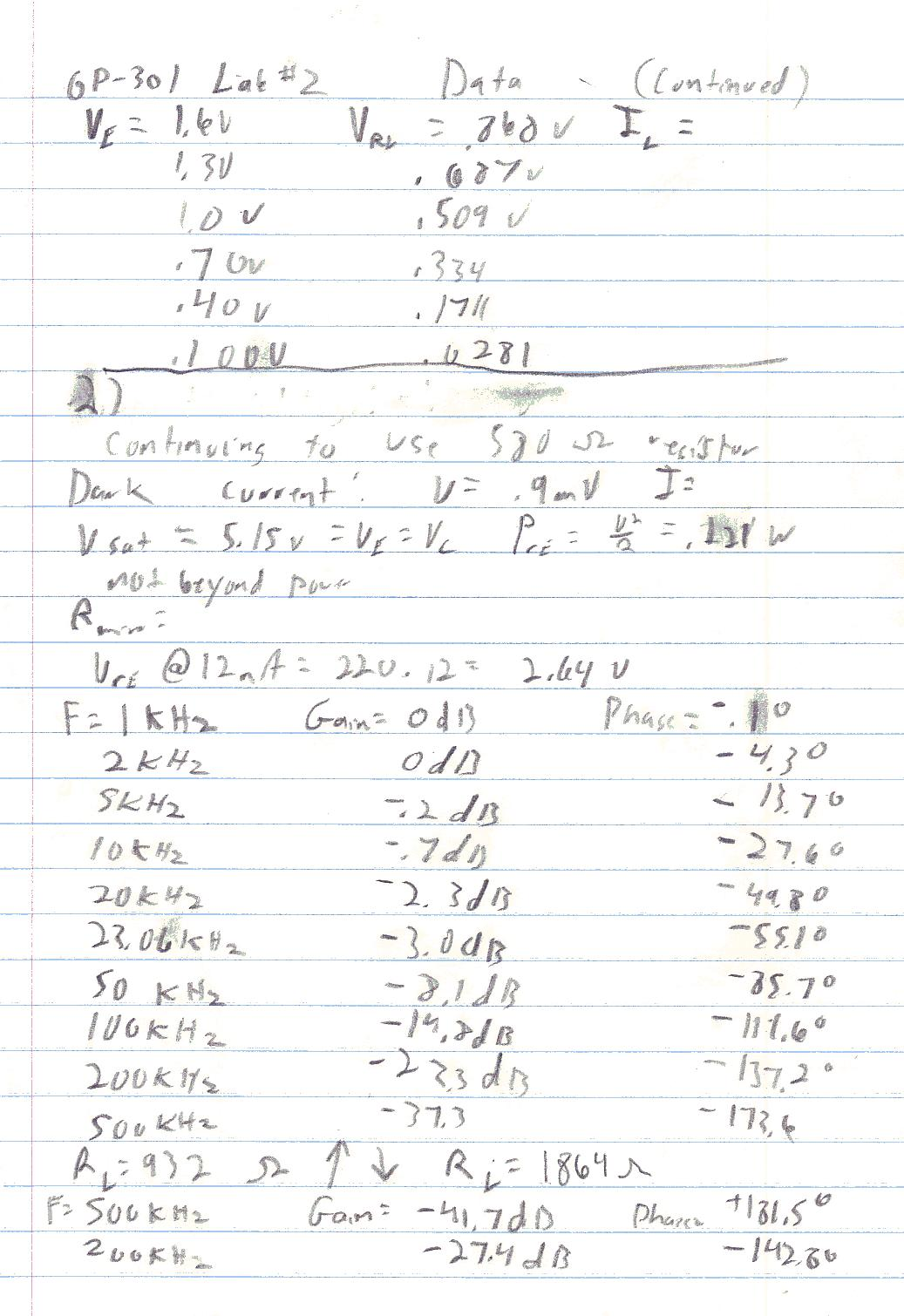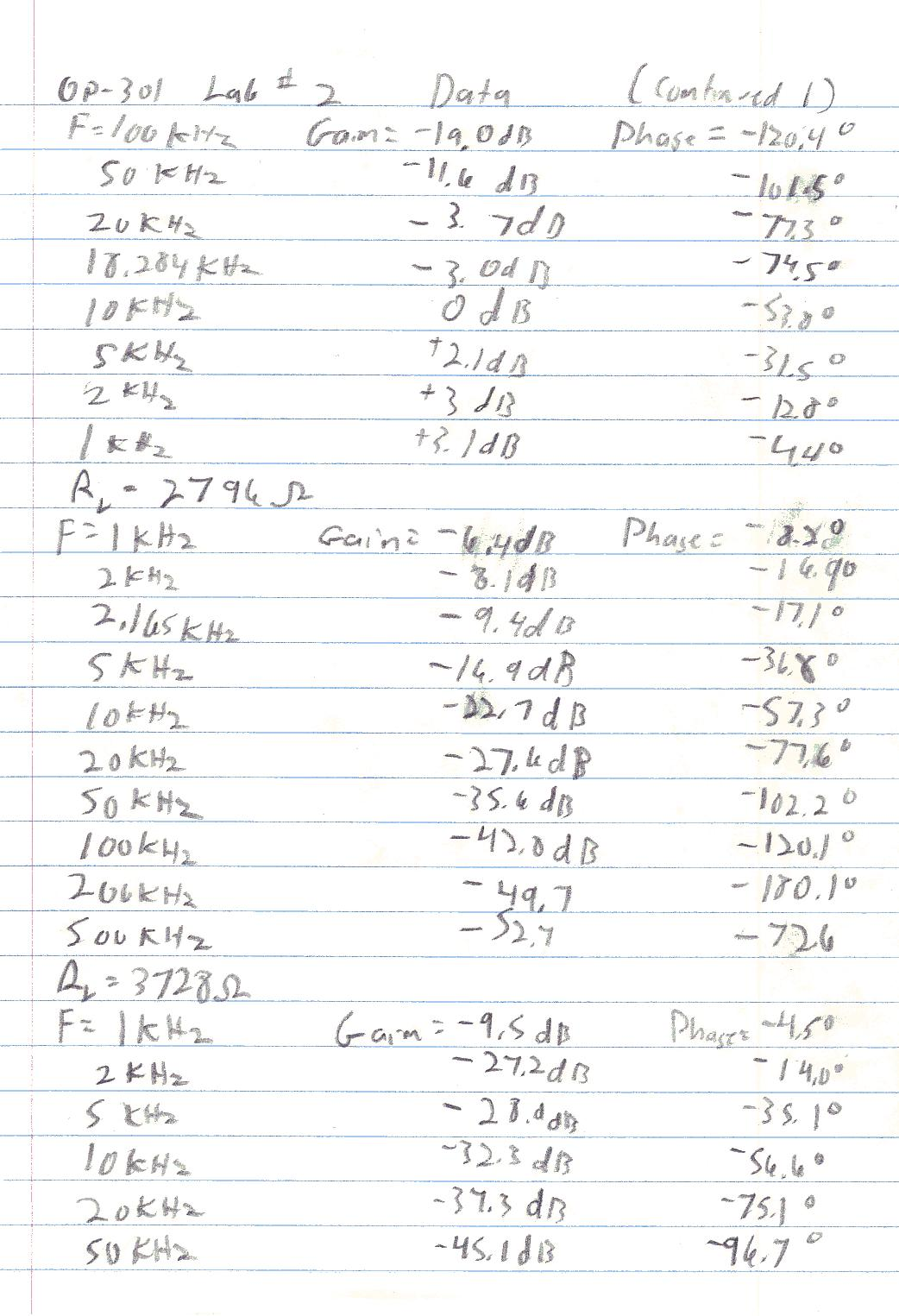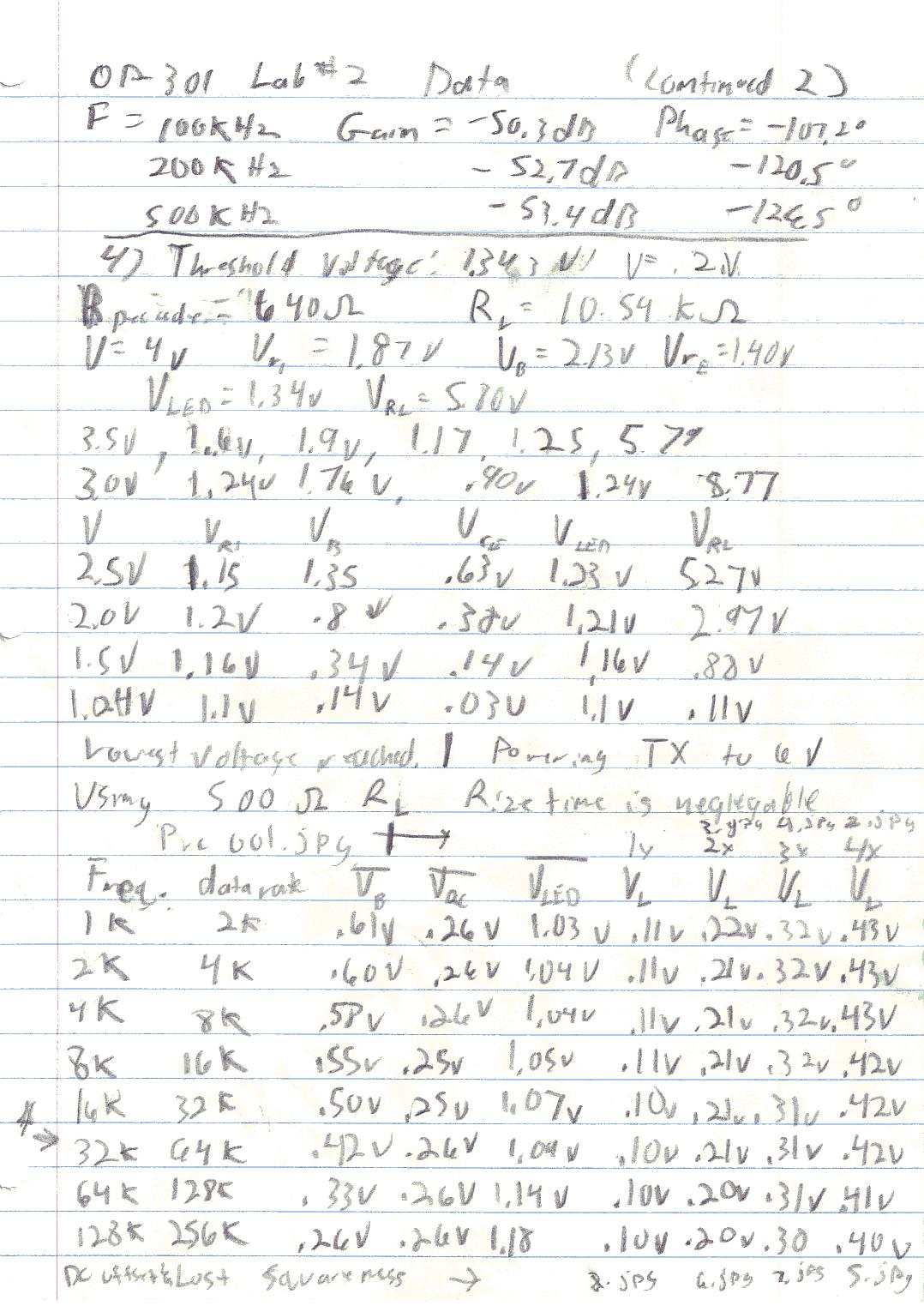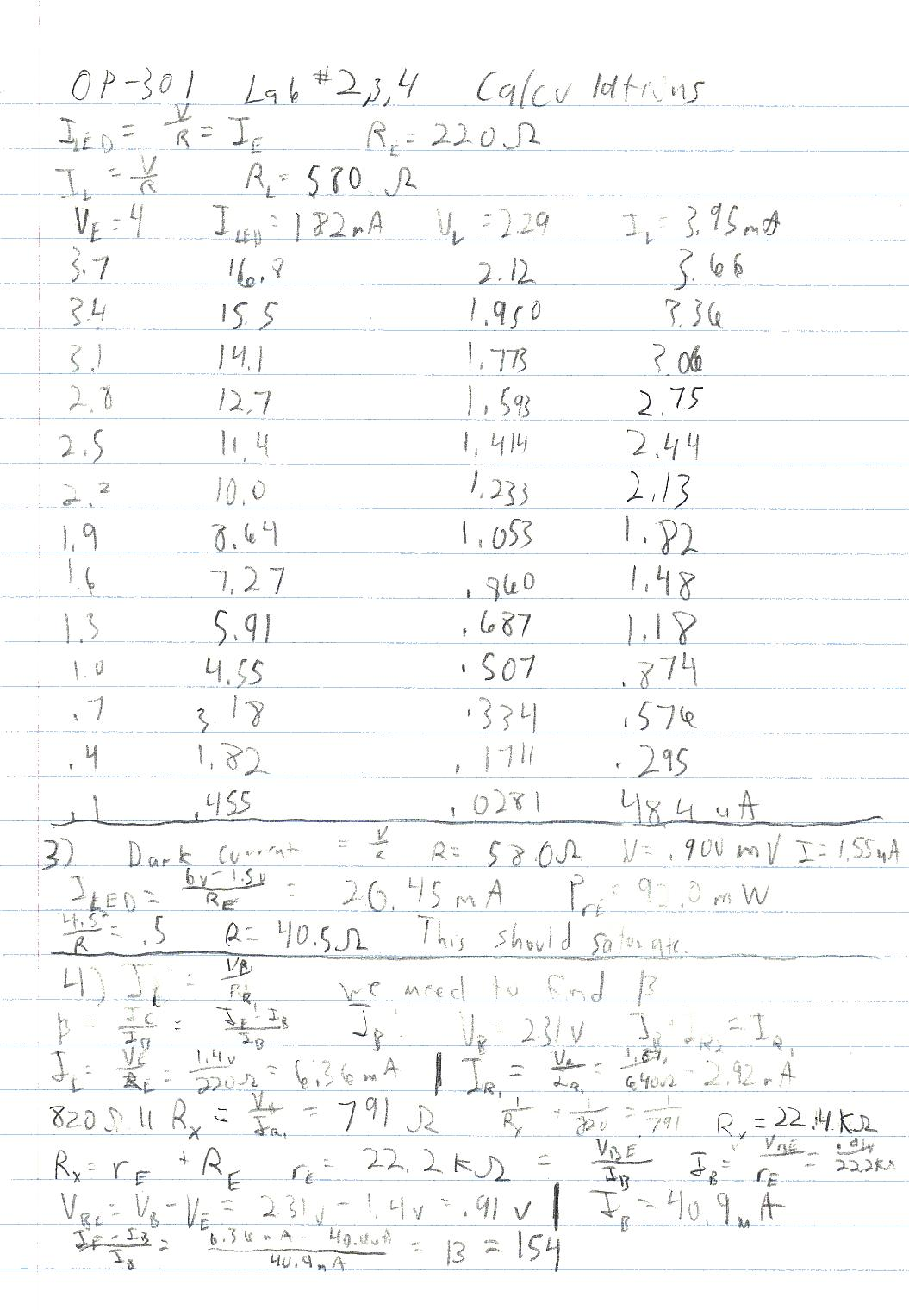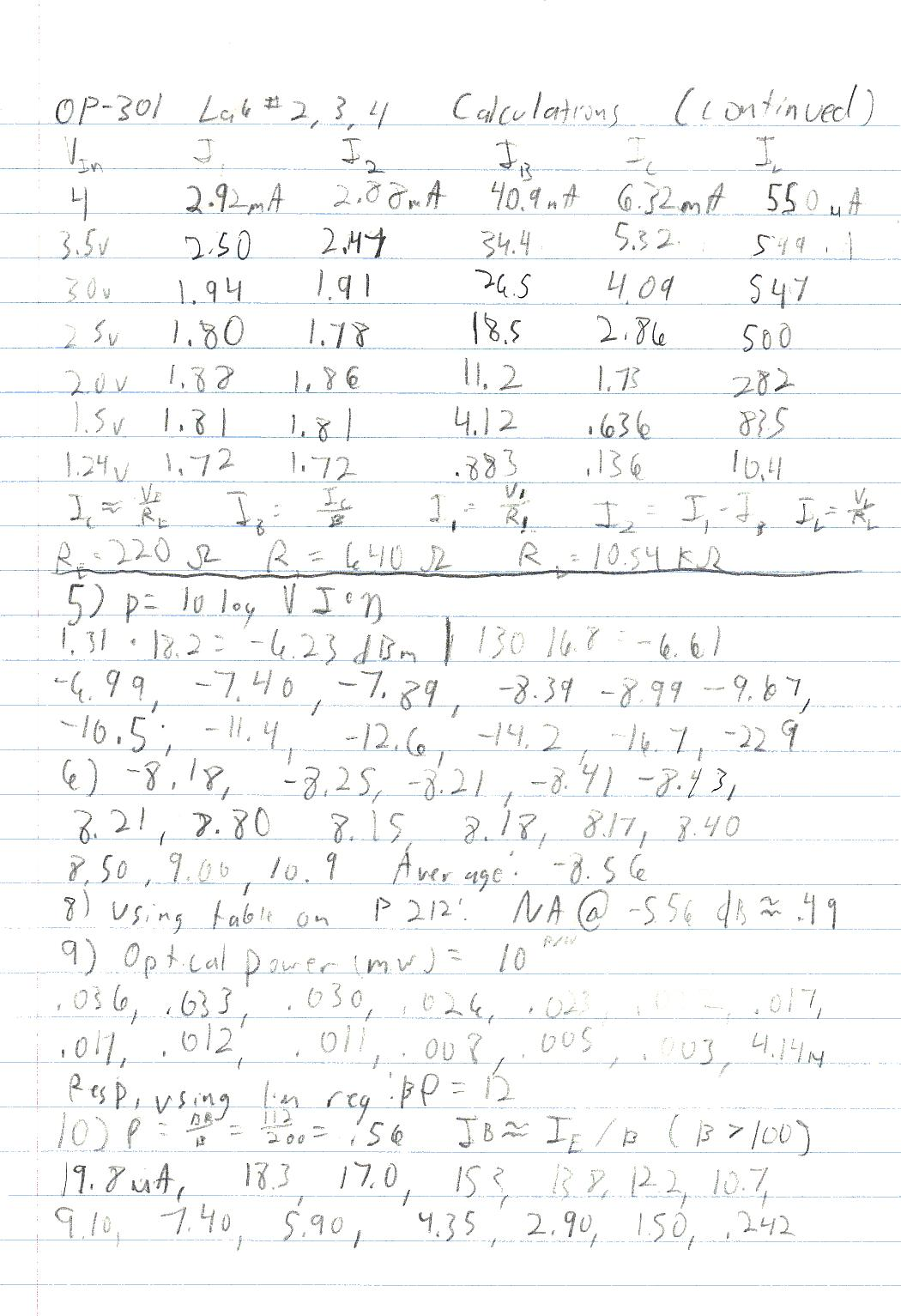OP - 301 / E01 spring 2002

Fiber Optic Communications

Professor: Weiler

Submitted By:

Andrew Buettner

Lab #2: Basic Optical Communications Link

Lab #3: Analog Optical Communications Link

Lab #4: Digital Optical Communication Link

Sunday, April 27, 2003

1) Cover Page 1

3) Objective 4

4) Components Used 4

5) Procedures 4

A) Basic Optical Communications 4

B) Analog Optical Communications 5

C) Digital Optical Communications 6

6) Lab Data / Results 6

1) Table 1 6

2) Diagram 1 7

3) Table 2 7

4) Diagram 2 8

5) Table 3 8

6) Table 4 8

7) Table 5 9

8) Table 6 9

9) Table 7 9

10) Table 8 10

11) Diagram 3 10

12) Table 9 10

13) Table 10 11

14) Table 11 11

15) Table 12 11

16) Diagram 4 12

17) Diagram 5 12

18) Diagram 6 13

19) Diagram 7 13

20) Diagram 8 14

21) Diagram 9 14

22) Diagram 10 15

23) Diagram 11 15

7) Answers to Lab Questions 15

8) Conclusions 21

9) Attachments 22Objective

The objective of this lab is to assemble a simple communications link to measure the various parameters involved in that communications link. Using the link, measurements of the volt-ampere relationship of the transmitter LED, the optical efficiency of the source, the quantum efficiency of the receiver, the responsivity of the detector, and the coupling loss of the link will be made. Then, an analog signal will be applied to the circuit and the resulting -3dB bandwidth of the signal will be measured, and calculated to form an r-c network associated with the receiving circuit. Also, the tradeoff between signal gain and bandwidth will be observed. The dark current for the photodetector, the transfer function for the overall system, the electrical bandwidth, and the photodetector rise time will also be calculated here. Lastly, the system will be reconfigured to handle a digital signal. The system's performance will be measured through rise times: The rise time will be defined as the time to transition form 10% power to 90% power. The calculated rise and fall times will be then compared against observed ones, using a square wave function generator.Components Used

1) Analog trainer #1982

2) Multimeter #1376

3) NOYES MLP 4-2 multimode light pack

4) Function generator #02-00004

5) Oscilloscope #01-00045

6) Gain – phase meter #09-00002

9) Various components from the OP-301 lab kitProcedures

A: Basic Optical Communications

1) Assemble the following circuit:2) Connect the fiber from the LED to the optical power meter.

3) Adjust R1 so that the voltage at the emitter is 4V.

4) Measure the voltage across the LED.

5) Measure the optical power from the LED.

6) Calculate the current through the LED.

7) Repeat steps 4 through 6 for emitter voltages of 3.7V, 3.4V, 3.1V, 2.8V, 2.5V, 2.2V, 1.9V, 1.6V, 1.3V, 1.0V, .7V, .4V, and .1V.

8) Connect the fiber optic cable to the remote side.

9) Set the emitter voltage back to 4V.

10) Adjust RL so that the voltage across it is at least 2V.

11) Measure the voltage across RL.

12) Calculate the current through RL.

13) Repeat steps 11 and 12 for emitter voltages of 3.7V, 3.4V, 3.1V, 2.8V, 2.5V, 2.2V, 1.9V, 1.6V, 1.3V, 1.0V, .7V, .4V, and .1V.

B: Analog Optical Communications

1) Assemble the following circuit:2) Power the detector circuit.

4) Measure, and calculate the current through RL, the dark current.

5) Remove power from the detector and power the transmitter side.

6) Saturate the transistor to allow the diode to emit maximum power.

7) Calculate the current through the LED assuming a voltage drop of 1.5V.

8) Calculate the power dissipated by RE, determine if it is beyond the rated power dissipation of ½ watt.

9) Calculate the minimum resistor value necessary to meet the ½ watt specification and determine if that value will allow saturation of the LED.

10) Power both the receiver and transmitter.

11) Adjust R1 so the current through the LED is between 10ma and 15ma.

12) Apply a 1Vpp, 1KHz sine wave to the input of the transmitter.

13) Observe the output of the receiver to ensure that it is an optimized sine wave, adjust RL if it is not.

14) Using the gain – phase meter, observe the signal loss through the link.

15) Repeat step 14 using sine waves of 2KHz, 5KHz, 10KHz, 20KHz, 50KHz, 100KHz, 200KHz, and 500KHz.

16) Make additional measurements to find the -3dB point as necessary.

17) Repeat steps 14 through 16 using double RL, triple RL, and quadruple RL.

C: Digital Optical Communications

1) Assemble the following circuit:2) Starting with RL of approximately 500W, and using a decade box to set R1 to 20KW, power the transmitter to 2V.

3) Power the receiver and adjust RL until the voltage across it is 3V.

4) Find the lowest transmitter voltage that produces a voltage drop across RL.

5) Set the transmitter voltage to 4V.

6) Record voltage across R1, base voltage, voltage across Rc, the voltage across the LED, and the voltage across RL.

7) Calculate the current through the decade box, the 820W resistor, base current, collector current, and current through RL.

8) Repeat step 6 and 7 for transmitter voltages of 3.5V, 3V, 2.5V, 2V, 1.5V, 1V, and .5V.

9) Remove the decade box from the circuit and connect the function generator.

10) Set the function generator to generate a 1KHz 4Vpp square wave.

11) Ensure that there is a signal visible across the load resistor, if not, increase the function generator's output voltage.

12) Measure the rise and fall time for the circuit.

13) Calculate the bit rate of the circuit.

14) Measure the average base voltage, the voltage across Rc, and the voltage across the LED.

15) Measure the voltage across RL.

16) Repeat steps 13 through 15 for frequencies of 1KHz, 2KHz, 4KHz, 8KHz, 16KHz, 32KHz, 64KHz, and 128KHz.

17) Using a double RL, triple RL and quadruple RL, repeat the RL measurement for the frequencies given in step 16.Lab Data / Results

1) Table 1: Transmitter Threshold

R1

RL

Threshold Voltage:

640W

10.54KW

1.343V

.2V

2) Diagram 1: Schematic 1 enlarged view3) Table 2: LED Power vs. Optical Power vs. Receiver Current:

VE

VLED

Optical Power

LED Current

4.0V

1.31V

-14.41dBm

18.2mA

2.29V

3.95mA

3.7V

1.30V

-14.86dBm

16.8mA

2.12V

3.66mA

3.4V

1.29V

-15.20dBm

15.5mA

1.950V

3.36mA

3.1V

1.29V

-15.81dBm

14.1mA

1.773V

3.06mA

2.8V

1.28V

-16.32dBm

12.7mA

1.593V

2.75mA

2.5V

1.27V

-16.60dBm

11.4mA

1.414V

2.44mA

2.2V

1.26V

-17.80dBm

10.0mA

1.233V

2.13mA

1.9V

1.25V

-17.82dBm

8.64mA

1.053V

1.82mA

1.6V

1.24V

-18.63dBm

7.27mA

.860V

1.48mA

1.3V

1.23V

-19.56dBm

5.91mA

.687V

1.18mA

1.0V

1.22V

-20.96dBm

4.55mA

.507V

0.87mA

.70V

1.20V

-22.68dBm

3.18mA

.334V

0.58mA

.40V

1.17V

-25.72dBm

1.82mA

.1711V

0.30mA

.10V

1.11V

-33.82dBm

0.46mA

.0281V

48.4mA

4) Diagram 2: Schematic 2 enlarged view: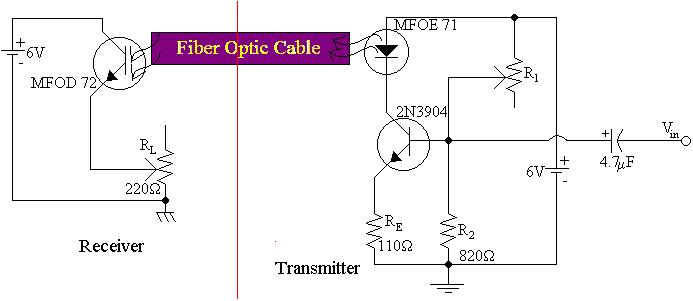5) Table 3: Saturation Voltage:

Voltage:

Current:

Resistance:

Power:

Will it Saturate?

1.5

20.45mA

220W

92.0mW

No

1.5

333mA

40.5W

500mW

Yes

.85V

142mA

220W

121mW

Yes

6) Table 4: Gain - Phase Observations using RL = 932W

Frequency

Gain

Phase

1KHz

0dB

-0.1o

2KHz

0dB

-4.3o

5KHz

-0.2dB

-13.7o

10KHz

-0.7dB

-27.6o

20KHz

-2.3dB

-49.8o

23.06KHz

-3.0dB

-55.1o

50KHz

-8.1dB

-85.7o

100KHz

-14.8dB

-111.6o

200KHz

-22.3dB

-137.2o

500KHz

-37.3dB

-173.6o

7) Table 5: Dark Current:

Dark Voltage:

Dark Current:

.9mV

1.55mA

8) Table 6: Gain - Phase Observations using RL = 1864W

Frequency

Gain

Phase

1KHz

+3.1dB

-4.4o

2KHz

+3dB

-12.8o

5KHz

+2.1dB

-31.5o

10KHz

0dB

-53.8o

18.284KHz

-3.0dB

-74.5o

20KHz

-3.7dB

-77.3o

50KHz

-11.6dB

-101.5o

100KHz

-19.0dB

-120.4o

200KHz

-27.4dB

-142.8o

500KHz

-41.7dB

+181.5o

9) Table 7: Gain - Phase Observations using RL = 2796W

Frequency

Gain

Phase

1KHz

-6.4dB

-8.2o

2KHz

-8.1dB

-16.9o

2.165KHz

-9.4dB

-17.1o

5KHz

-16.9dB

-13.8o

10KHz

-22.7dB

-57.3o

20KHz

-27.6dB

-77.6o

50KHz

-35.6dB

-102.2o

100KHz

-42.8dB

-120.1o

200KHz

-49.7dB

-180.1o

500KHz

-52.7dB

-72.6o

10) Table 8: Gain - Phase Observations using RL = 3728W

Frequency

Gain

Phase

1KHz

-9.5dB

-4.5o

2KHz

-27.2dB

-14.0o

5KHz

-28.9dB

-35.1o

10KHz

-32.3dB

-56.6o

20KHz

-37.3dB

-75.1o

50KHz

-45.1dB

-96.7o

100KHz

-50.3dB

-107.2o

200KHz

-52.7dB

-120.5o

500KHz

-53.4dB

-126.5o

11) Diagram 3: Schematic 3 enlarged view: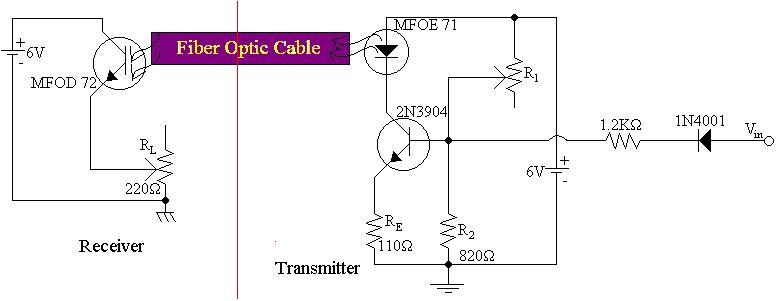12) Table 9: Transmitter Input Voltage Table (Voltage):

Vin

VR1

VB

VRE

VLED

VL

4.0V

1.87V

2.13V

1.40V

1.34V

5.80V

3.5V

1.60V

1.90V

1.17V

1.25V

5.79V

3.0V

1.24V

1.76V

.90V

1.24V

5.77V

2.5V

1.15V

1.35V

.63V

1.23V

5.27V

2.0V

1.2V

.80V

.38V

1.21V

2.97V

1.5V

1.16V

.34V

.14V

1.16V

.88V

1.24V

1.1V

.14V

.03V

1.1V

.11V

13) Table 10: Transmitter Input Voltage Table (Current):

Vin

I1

I2

IB

IC

IL

4.0V

2.92mA

2.88mA

40.9mA

6.32mA

550mA

3.5V

2.50mA

2.47mA

34.4mA

5.32mA

549mA

3.0V

1.94mA

1.91mA

26.5mA

4.09mA

547mA

2.5V

1.80mA

1.78mA

18.5mA

2.86mA

500mA

2.0V

1.88mA

1.86mA

11.2mA

1.73mA

282mA

1.5V

1.81mA

1.81mA

4.12mA

.636mA

83.5mA

1.24V

1.72mA

1.72mA

.883mA

.136mA

10.4mA

14) Table 11: Average Voltages for Digital Transmission:

Frequency:

Data Rate:

VB

VRC

VLED

VL

2*VL

3*VL

4*VL

1KHz

2Kbps

.61V

.26V

1.03V

.11V

.22V

.32V

.43V

2KHz

4Kbps

.60V

.26V

1.04V

.11V

.21V

.32V

.43V

4KHz

8Kbps

.58V

.26V

1.04V

.11V

.21V

.32V

.43V

8KHz

16Kbps

.55V

.25V

1.05V

.11V

.21V

.32V

.42V

16KHz

32Kbps

.50V

.25V

1.07V

.10V

.21V

.31V

.42V

32KHz

64Kbps

.42V

.26V

1.09V

.10V

.21V

.31V

.42V

64KHz

128Kbps

.33V

.26V

1.14V

.10V

.20V

.31V

.41V

128KHz

256Kbps

.26V

.26V

1.18V

.10V

.20V

.30V

.40V

15) Table 12: Rise Time for signals

1.0KW

1.5KW

2KW

500W

Rise Time:

negligible

negligible

negligible

negligible

16) Diagram 4: Rise time signal for RL = 500W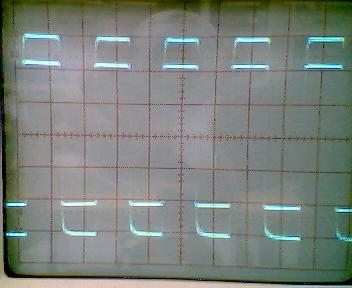17) Diagram 5: Rise time signal for RL = 1KW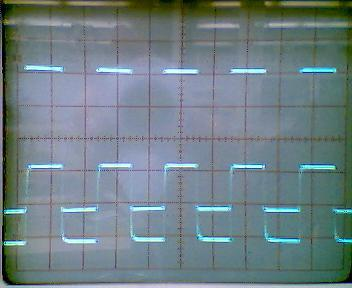18) Diagram 6: Rise time signal for RL = 1.5KW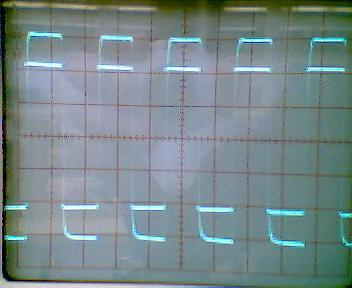19) Diagram 7: Rise time signal for RL = 2KW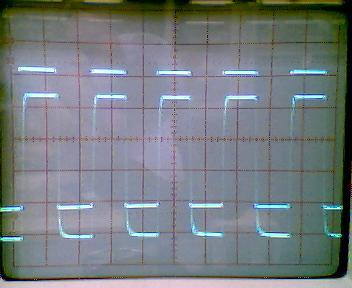20) Diagram 8: Maximum Data Rate signal for RL = 500W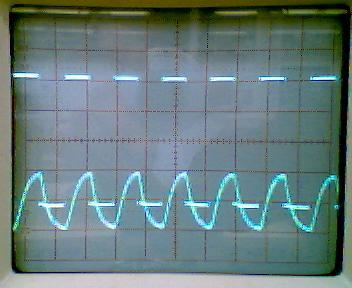21) Diagram 9: Maximum Data Rate signal for RL = 1KW22) Diagram 10: Maximum Data Rate signal for RL = 1.5KW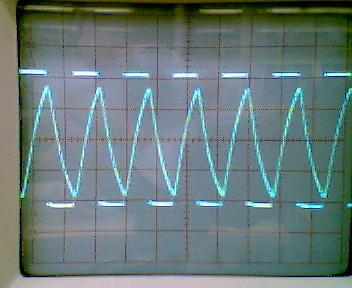23) Diagram 11: Maximum Data Rate signal for RL = 2KWAnswers to Lab Question

1) Q: What wavelength does the LED emit?

A: 1310nM

2) Q: Plot ID vs. VD for the LED, what is the turn-on voltage?.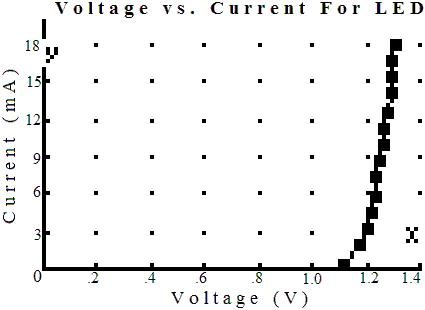3) Q: Using the answer to Q1, what material is the LED made of?

A: InGaAs

4) Q: Plot the LED's optical power vs. forward current.

A: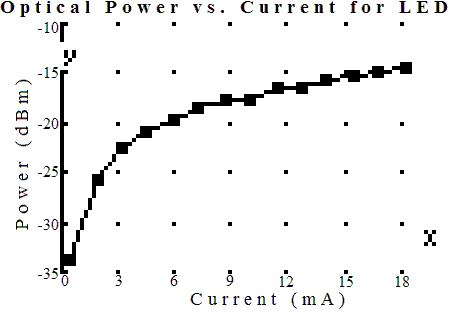5) Q: Using an assumed efficiency of 1%, calculate and plot the expected optical power output of the LED, and overlay it with the existing plot.

A: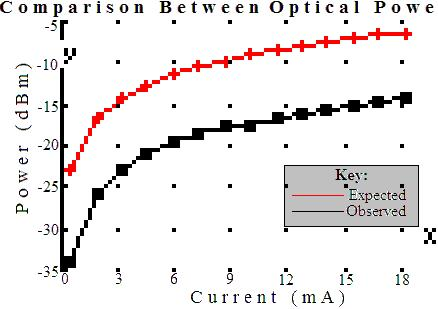6) Q: Calculate the difference, in dB, for the data in Q5.

A: Power Output

Observed:

Expected:

Difference:

-14.41dBm

-6.23dBm

-8.18dB

-14.86dBm

-6.61dBm

-8.25dB

-15.20dBm

-6.99dBm

-8.21dB

-15.81dBm

-7.40dBm

-8.41dB

-16.32dBm

-7.89dBm

-8.43dB

-16.60dBm

-8.83dBm

-8.21dB

-17.80dBm

-9.00dBm

-8.80dB

-17.82dBm

-9.67dBm

-8.15dB

-18.63dBm

-10.5dBm

-8.18dB

-19.56dBm

-11.4dBm

-8.17dB

-20.96dBm

-12.6dBm

-8.40dB

-22.68dBm

-14.2dBm

-8.50dB

-25.72dBm

-16.7dBm

-9.00dB

-33.82dBm

-22.9dBm

-10.9dB

Average:

-8.56dB

7) Q: Assuming a 3dB connection loss, what, then, is the coupling loss of the system?

A: -5.56dB

8) Q: Assuming the LED is a Lambertain source, what is the NA of the fiber?

A: NA Z .49

9) Q: Plot the optical power vs. the load current, what is the responsivity of the detector?

A: br Z 112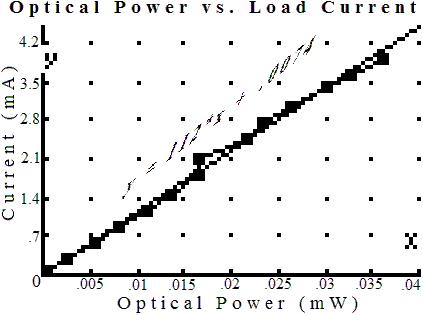10) Q: Assuming the phototransistor has a b of 200, calculate r for the base of the transistor, and show the base current for Table 2.

A: r Z .56

Power is in dB Current is in mA

Pi

-14.4

-14.9

-15.2

-15.8

-16.3

-16.6

-17.8

-17.8

-18.6

-19.6

-21.0

-22.7

-25.7

-33.8

IB

19.8

18.3

17.0

15.3

13.8

12.2

10.7

9.10

7.40

5.90

4.35

2.90

1.50

.242

11) Q: Calculate the quantum efficiency of the photodiode.

A: h Z .541

12) Q: Plot the transfer function for all four values of RL.

A: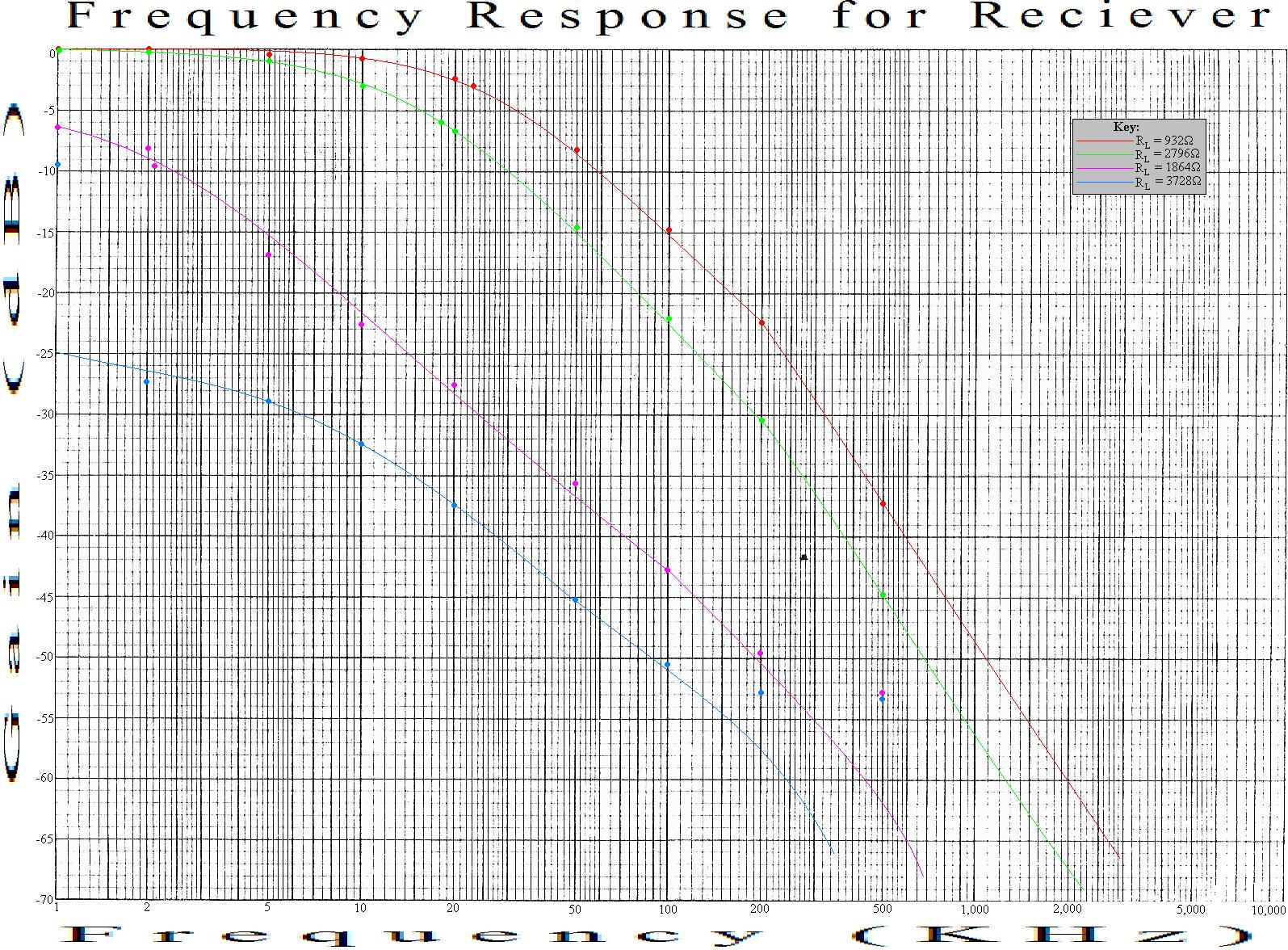13) Q: Using the -3dB point, calculate the capacitance of the photodetector, and the rise time, also show the phase.

A: Average Capacitance: 89.1mF

Resistance:

932W

1864W

2796W

3782W

-3dB Point:

23.06KHz

5.01KHz

2.165KHz

7KHz

Capacitance:

46.5mF

107mF

165mF

37.8mF

Phase Angle:

-55.1o

-74.5o

-17.1o

-45.9o

Rise-Time

.0952s

.438s

1.01s

.314s

14) Q: If the saturation voltage of the phototransistor is .4V and the maximum power dissipation is 150mW, what is the maximum load current, and minimum RL? What, then would be the maximum bandwidth?

A: IL = 2.67A; RL = 2.1W; Infinite bandwidth

15) Q: Plot RL vs. the -3dB bandwidth. What would the bandwidth be for RL = 1KHz? Also, plot the peak output voltage.

A: BW Z 9.5KHz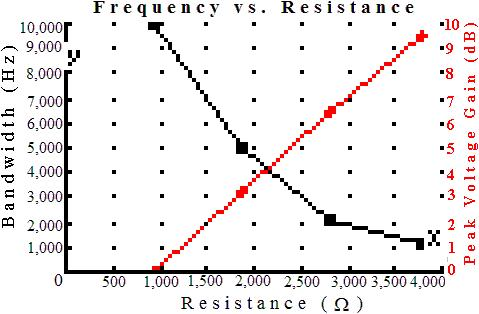16) Q: What would be the optimal load resistance?

A: Optimal Resistance: 2.2KW

17) Q: What would be the expected output voltage , relative to input voltage, and bandwidth for the resistance selected in Q16?

A: Gain : 4dB Bandwidth: 4KHz

18) Q: What is the minimum transmitter voltage that will produce an output signal?

A: 1.343V

19) Q: What voltage would be required at the input of schematic 3 to saturate the LED?

A: Vsat = 5.56V

20) Q: When the LED is saturated by this voltage, how much current flows through it?

A: ILED = 21.1mA

21) Q: What is the b of the transistor?

A: b = 154

22) Q: What are the rise and fall times for each RL?

A: Rise and fall times were negligible, and scope was not able to illustrate them.

23) Q: Based on the rise time estimates, what is the capacitance of the photodiode?

A: Insufficient data to perform calculation. Capacitance is < 100nF.

24) Q: How does this capacitance compare to the one discovered in Q13?

A: The capacitances are totally different.

25) Q: What correlation, if any, exist between the bandwidth and the rise time?

A: There is no correlation because the bandwidth is more determined on the ability for the system to hold the voltage for sampling more than transition time.

26) Q: What is the maximum NRZ for each load resistor?

A:

Resistance:

500W

1KW

1.5KW

2KW

Maximum Data Rate:

64Kbps

64Kbps

64Kbps

64Kbps

27) Q: If 1V were required at the load, what would be the maximum NRZ data rate for the system?

A: 64KbpsConclusionsAttachments

1. Original lab handout

2. Original lab data

3. Calculations